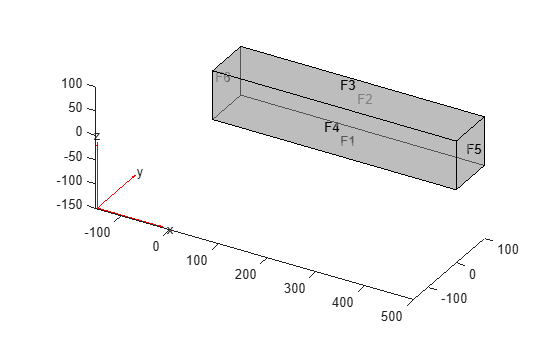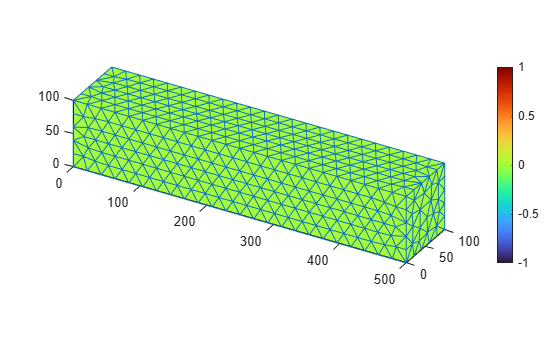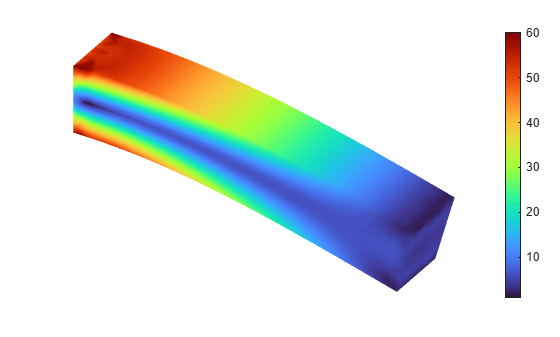pdeviz

Create and plot PDE visualization object

Description

example

pdeviz(MeshData,NodalData) creates a PDEVisualization object and plots the data at the mesh nodes as a surface plot. For details, see PDEVisualization Properties.

example

pdeviz(MeshData) creates a PDEVisualization object and plots the mesh.

example

pdeviz(___,Name,Value) customizes the plot appearance using one or more Name,Value arguments. Use name-value arguments with any combination of arguments from the previous syntaxes.

pdeviz(figure,___) specifies the graphics container for the PDEVisualization object. For example, you can plot the object in Figure 3 by specifying pdeviz(figure(3), ___).

example

V = pdeviz(___) returns a handle to the PDEVisualization object, using any of the previous syntaxes.

Examples

collapse all

Use the pdeviz function to create a PDE visualization object and plot it. Change the properties of this object to interact with the resulting plot.

Create a structural analysis model for a 3-D problem.

structuralmodel = createpde('structural','static-solid');

Import the beam geometry and plot it.

importGeometry(structuralmodel,'SquareBeam.stl');
pdegplot(structuralmodel,'FaceLabels','on','FaceAlpha',0.5)Specify the Young's modulus and Poisson's ratio.

structuralProperties(structuralmodel,'PoissonsRatio',0.3, ...
'YoungsModulus',210E3);

Specify that face 6 is a fixed boundary.

structuralBC(structuralmodel,'Face',6,'Constraint','fixed');

Specify the surface traction for face 5.

'SurfaceTraction',[0;0;-2]);

Generate a mesh and solve the problem.

msh = generateMesh(structuralmodel);
structuralresults = solve(structuralmodel);

Call pdeviz with only the mesh data. This call creates a PDEVisualization object and plots the mesh.

figure
v = pdeviz(msh)v =
PDEVisualization with properties:

MeshData: [1x1 FEMesh]
NodalData: [0x1 double]
MeshVisible: on
Transparency: 1
Position: [0.1300 0.1100 0.6561 0.8150]
Units: 'normalized'

Show all properties

Update the plot by adding the von Mises stress as the NodalData property of the PDEVisualization object v. The plot now shows the von Mises stress and the mesh.

figure
v.NodalData = structuralresults.VonMisesStress;

Update the plot by adding the displacement as the DeformationData property of the PDEVisualization object v. The plot shows the deformed shape with the von Mises stress.

figure
v.DeformationData = structuralresults.Displacement;Update the plot to hide the mesh.

figure
v.MeshVisible = 'off';Update the plot to show the axes.

figure
v.AxesVisible = 'off';Input Arguments

collapse all

Finite element mesh, specified as an FEMesh Properties object.

Data at mesh nodes, specified as a column vector.

Data Types: double

Name-Value Arguments

Specify optional comma-separated pairs of Name,Value arguments. Name is the argument name and Value is the corresponding value. Name must appear inside quotes. You can specify several name and value pair arguments in any order as Name1,Value1,...,NameN,ValueN.

Example: pdeviz(model.Mesh,results.NodalSolution,'MeshVisible','on','DeformationData',results.Displacement)

Surface transparency, specified as a real number from 0 through 1. The default value 1 indicates no transparency. The value 0 indicates complete transparency.

Data Types: double

Toggle to show mesh, specified as 'on' or 'off', or as numeric or logical 1 (true) or 0 (false). A value of 'on' is equivalent to true, and 'off' is equivalent to false. Thus, you can use the value of this argument as a logical value.

When plotting only the mesh, the default is 'on'. Otherwise, the default is 'off'.

Data Types: char | string

Mesh deformation data, specified as one of the following:

• An FEStruct object with the properties ux, uy, and, for a 3-D geometry, uz

• A structure array with the fields ux, uy, and, for a 3-D geometry, uz

• A matrix with either two columns for a 2-D geometry or three columns for a 3-D geometry

Level of mesh deformation, specified as a nonnegative number. Use this name-value argument together with DeformationData.

pdeviz computes the default value of DeformationScaleFactor based on the mesh and the value of DeformationData.

Data Types: double

Toggle to hide or show axes, specified as 'on' or 'off', or as numeric or logical 1 (true) or 0 (false). A value of 'on' is equivalent to true, and 'off' is equivalent to false. Thus, you can use the value of this argument as a logical value.

Background color, specified as an RGB triplet, a hexadecimal color code, a color name, or a short name.

Output Arguments

collapse all

Visualization container, returned as a handle to the PDEVisualization object. For details, see PDEVisualization Properties.